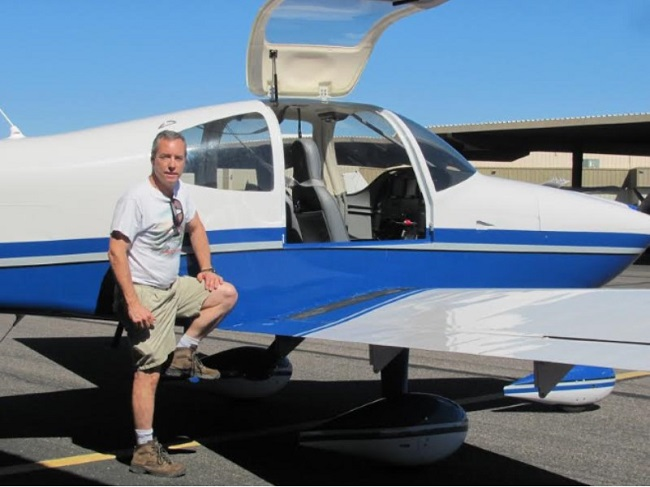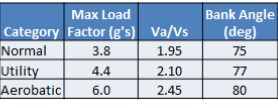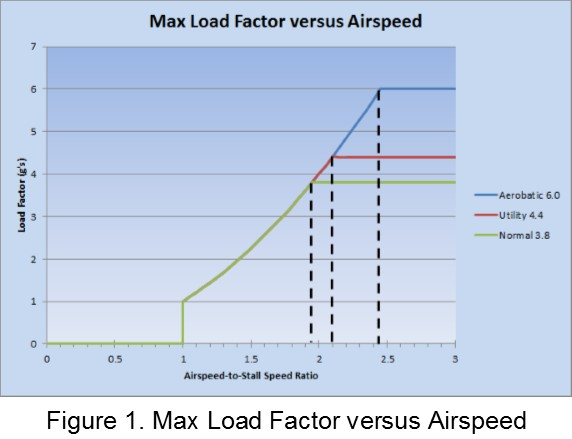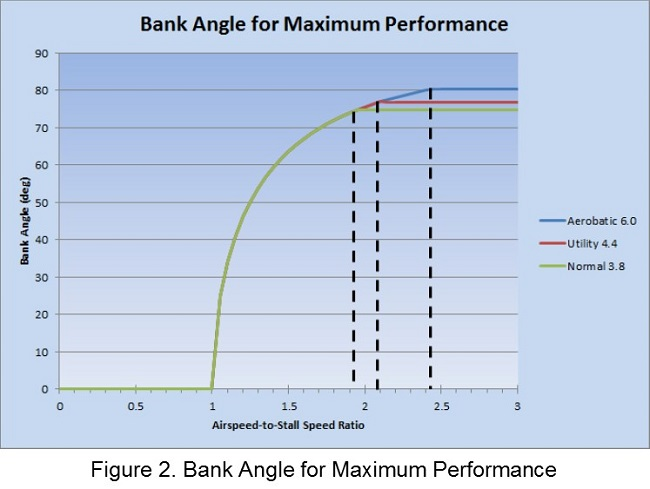MANEUVERING SPEEDS

By Mike Andresen

Most things you read about regarding maneuvering speed address the bending of an airplane aspect of it, but there is another aspect to maneuvering speed that I'm going to discuss in this article. You are about to learn why it is called maneuvering speed and not called bending-an-airplane speed.

Recall that for an aircraft to maintain constant altitude, the vertical component of lift must equal the weight of the airplane plus the tail down force. For the purpose of keeping this discussion simple, I will use weight to mean weight plus tail down force. For the airplane to turn, the aircraft must generate a horizontal component of lift by increasing the overall lift generated such that the vertical component of lift maintains the weight of the aircraft and the horizontal component of lift causes the aircraft to turn. This is accomplished by either increasing the angle of attack or by increasing airspeed. Let's assume power is constant and the turn is made by increasing control back pressure to increase angle of attack.As the airplane is in the constant altitude turn, the wing is generating lift in excess of the weight. The ratio of total lift to weight is known as the load factor. In a sixty degree bank, the wing will have to generate twice the weight of the airplane in order for the vertical component of lift to equal weight. Under this condition, the load factor is two and the pilot will feel twice the force of gravity which is referred to as 2G. Figure 1 is a graph of load factor versus airspeed. In the graph, airspeed is expressed as a multiple of the stall speed. The maneuvering speed is strictly a function of the stall speed and maximum load factor of the airplane. For a normal category airplane where the maximum positive load factor is 3.8G's, the maneuvering speed is always 1.95 times the stall speed.Each load factor is achieved by maintaining constant altitude with the airplane in a specific bank angle. Figure 2 is a graph of maximum bank angle achievable versus airspeed. This graph tells you that in order to perform the 45 degree bank, the 1.4G turn required for private pilot test standards, the airspeed must be at least 1.2 times the stall speed. It also tells us that the maximum bank angle achievable in a normal category airplane is 75 degrees. Parachute required! Flying at the maximum possible bank angle will result in the fastest turn rate and the minimum turn radius possible.The point of all this is that the maximum performance turn is achieved in an airplane when flying at maneuvering speed with a maximum supported bank angle. For a normal category airplane, this means flying at 1.95 times the stall speed in a 75 degree bank pulling 3.8G's. That is quite a bit of performance that we can get from our airplanes.

To summarize, at any speed below maneuvering speed turning at the airplane’s minimum turn radius is possible only by increasing the turning rate and g-load to the verge of an accelerated stall. Above maneuvering speed, you are g-limited by your category and hence cannot increase turn rate to achieve minimum turn radius.

Now that you have reached your cruise altitude, you can switch off the fasten seatbelt sign and take a moment to relax. If you are in a technologically advanced airplane, or carrying a tablet, you are probably looking at multi-colored LCDs throwing all sorts of information at you. My Electronic Flight Information System has readouts of fuel flow, range, miles-per-gallon and indicated/true/ground speeds. Is there a way to minimize fuel flow, maximize MPG, and maximize airspeed all at once? Probably not, but we will explore the factors effecting each and some other performance metrics that we may not normally think about.

Maximum Time Aloft

The airplane's time aloft or endurance is its fuel on board (gal) divided by fuel flow (gph). To maximize time aloft, one must maximize fuel capacity and minimize fuel flow. Fuel flow is minimized by using the least amount of power to sustain flight. This is the bottom of the power required for level flight curve. On an endurance flight, as fuel is consumed and the airplane weight decreases the minimum power required will decrease and the throttle can be reduced. To fly for maximum endurance, you will be flying really slow, at or near your Vx speed, so this is rarely done in general aviation. An example of when this would be useful is on an observation mission where the airplane needs to remain on station for the longest possible time.

Best Miles per Gallon, Best Range Speed

This probably gets your attention because now we are talking about saving money. The best miles per gallon will result in the maximum range of the airplane. Another way to express it is that this cruise speed will use the least amount of fuel for a given trip distance. The fuel used on a trip depends on fuel flow and the time it takes to make the trip. So now we need to minimize fuel flow (keep power low) but make the trip fast enough to use as little fuel as possible (keep power high!). The middle ground is found at the bottom of the L/D curve of the airplane and is close to, if not the same as, the Vy speed. In the interest of saving fuel, we get to fly a little faster but still at a relatively slow airspeed.

Best Speed per Gallon

Optimum cruise speed was derived by B.H. Carson of the U.S. Naval Academy in 1980. For those of you that can remember back that far, this was after the oil embargo. Suddenly the country was focused on the fuel efficiency of cars and airplanes. Carson addressed the question of using miles-per-gallon as the optimizing metric for airplanes. He noted that for airplanes the speed for optimum fuel efficiency was quite slow and utilized only a small percentage of the airplane's available horse power. Instead he derived a new metric, the optimum cruise speed which maximizes the speed of the airplane relative to fuel flow. It is the best speed per gallon-per-hour ratio that can be achieved. The optimum cruise speed that he derived is 1.32 times the best miles-per gallon speed. It is a bit more practical than flying at best range speed.

Here are the V speeds discussed in this article derived from the performance charts I measured during the flight test period of my RV-10. The blue line is the thrust required for level flight and is read from the axis on the left in pounds. The red line is the power required for level flight and is read from the vertical axis on the right in horsepower. The maximum endurance speed is a very slow 70 KIAS and only requires 57 horsepower from the 260 horsepower engine. The maximum range speed is 90 KIAS and requires about 100 horsepower. The optimum cruise speed is 120 KIAS, still somewhat slow, but better, and requires around 120 horse power - still only 46% power.

Next month we will discuss how to determine these performance curves for an airplane through flight testing.